Ch 2. Fluid Statics Multimedia Engineering Fluids PressureVariation PressureMeasurement HydrostaticForce(Plane) HydrostaticForce(Curved) Buoyancy
 Chapter 1. Basics 2. Fluid Statics 3. Kinematics 4. Laws (Integral) 5. Laws (Diff.) 6. Modeling/Similitude 7. Inviscid 8. Viscous 9. External Flow 10. Open-Channel Appendix Basic Math Units Basic Equations Water/Air Tables Sections Search eBooks Dynamics Fluids Math Mechanics Statics Thermodynamics Author(s): Chean Chin Ngo Kurt Gramoll ©Kurt GramollFLUID MECHANICS - EXAMPLES Question 1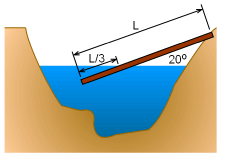Telephone Pole Partially Submerged in a Lake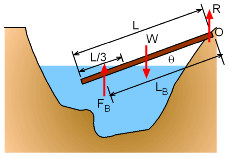Problem Diagram A wooden telephone pole paritally falls into a lake as shown. What is the density of the pole? Assume the density of the water is 1.94 slug/ft3. Solution 1 The pole will come to a static position where the sum of the moments about the point O is equal to zero.      ΣMo = 0 Therefore, the buoyancy force multiplied by its lever arm must be equal and opposite the gravitational force times its lever arm.      ΣMo = 0 = (WpoleLpole - FBLB)cosθ where Lpole = L/2 (gravity acts uniformly along the entire length), and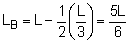(buoyancy force acts at the centroid of the submerged part of the pole). Note, θ is not needed since it factors out of the equation. Summing moments at O gives,      WpoleLpole = FBLB where      Wpole = ρpole V g = ρpole (π r2 L) g      FB = ρwater V g = ρwater (π r2 L/3) g thus,       ρpole (π r2 L) g (L/2) = ρwater (π r2 L/3) g (5L/6) Cancelling terms,      ρpole (1/2) =(1.94 slug/ft3) (5/18) The density of the pole is      ρpole = 1.078 slug/ft3 Question 2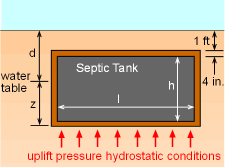Septic Tank Septic tanks are used by homes in rural areas not serviced by sanitary sewers. Assume the tank is 6 ft x 6 ft x 10 ft (long) with 4 in. concrete walls, and assume 1 ft of soil over the tank. At what groundwater depth, d, if any, will the tank float? Any recommendations to keep it from floating? Assume γconcrete = 150 pcf, γsoil = 120 pcf, and γwater = 62.4 pcf Solution 2 Inside dimensions: h, w, l Outside dimensions: h + 2t, w + 2t, l + 2t where t is the wall thickness      Vc = (h + 2t)(w + 2t)(l + 2t) - hwl      A = (w + 2t)(l + 2t) Since there is 1 ft of soil over the top of tank,      z = (1 ft) + (h +2t) - d where d is the water table.      γconcrete = 150 pcf      γsoil = 120 pcf      γwater = 62.4 pcf To float, the buoyancy force acting on the bottom of the tank must be greater than the weight of the tank and the soil on top.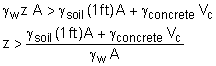septic tank: h = 6 ft, w = 6 ft, l = 10 ft, t = 4 in.      Vc = 474.1 - 360 = 114.1 ft3      A = 71.11 ft2      z = 7.67 - d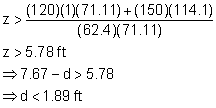If the groundwater table depth is less than 1.89 ft, the septic tank will float. Recommendation to keep it from floating: use 6 in. walls instead of 4 in.

Practice Homework and Test problems now available in the 'Eng Fluids' mobile app
Includes over 250 problems with complete detailed solutions.
Available now at the Google Play Store and Apple App Store.